7417

Physics Force & Laws Of Motion Level: Misc Level

You want to enlarge a skating surface so you stand on the ice at one end and aim a hose horizontally to spray water on the schoolyard pavement. Water leves the hose at 3. 9 kg/s with a speed 19. 8 m/s. If your mass is 81 kg, what is your recoil acceleration? Neglect friction and the mass of the hose. Answer in units of m/s squared.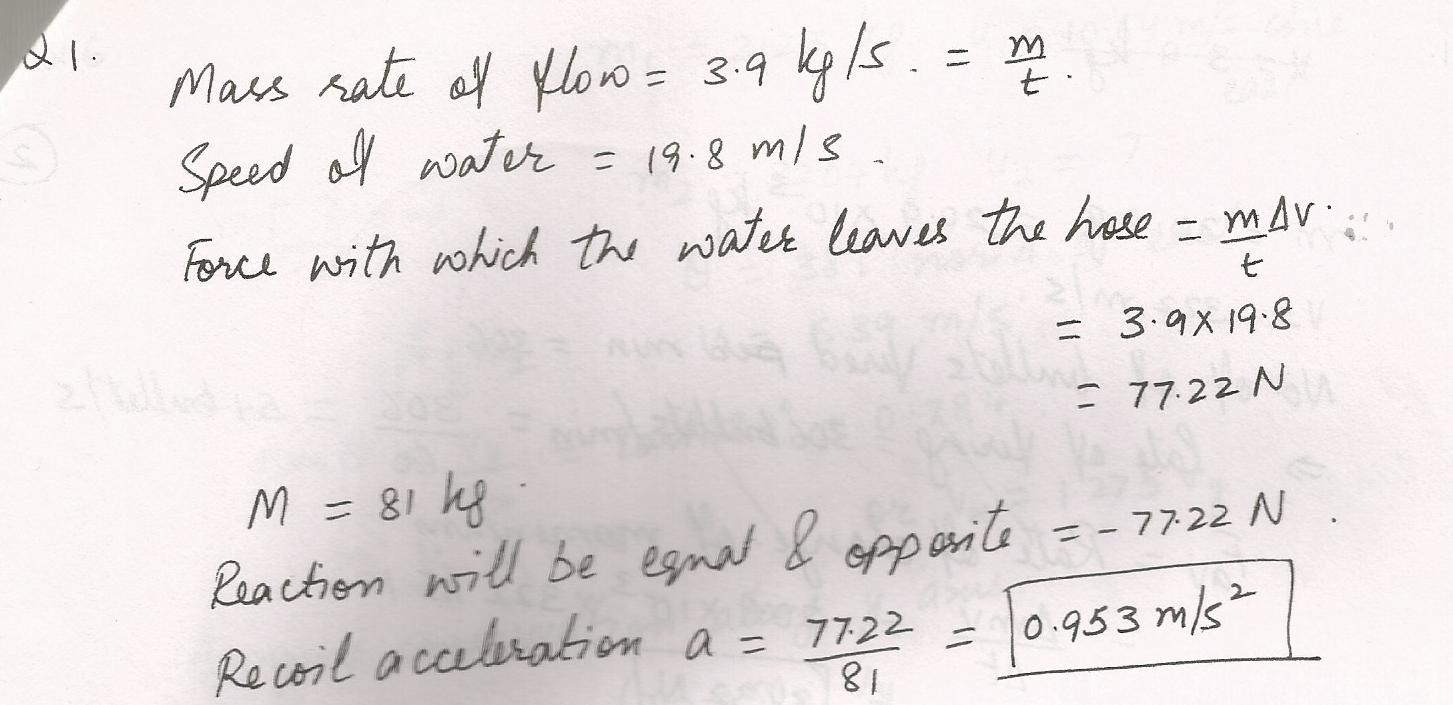7406

Physics Force & Laws Of Motion Level: Misc Level

A hanging sign which weighs 50 N is pished out from the wall by a bracket, and supported by a rope tied to the wall.The rope makes an angle of 30 degrees with the wall. Find the force of tention in the rope.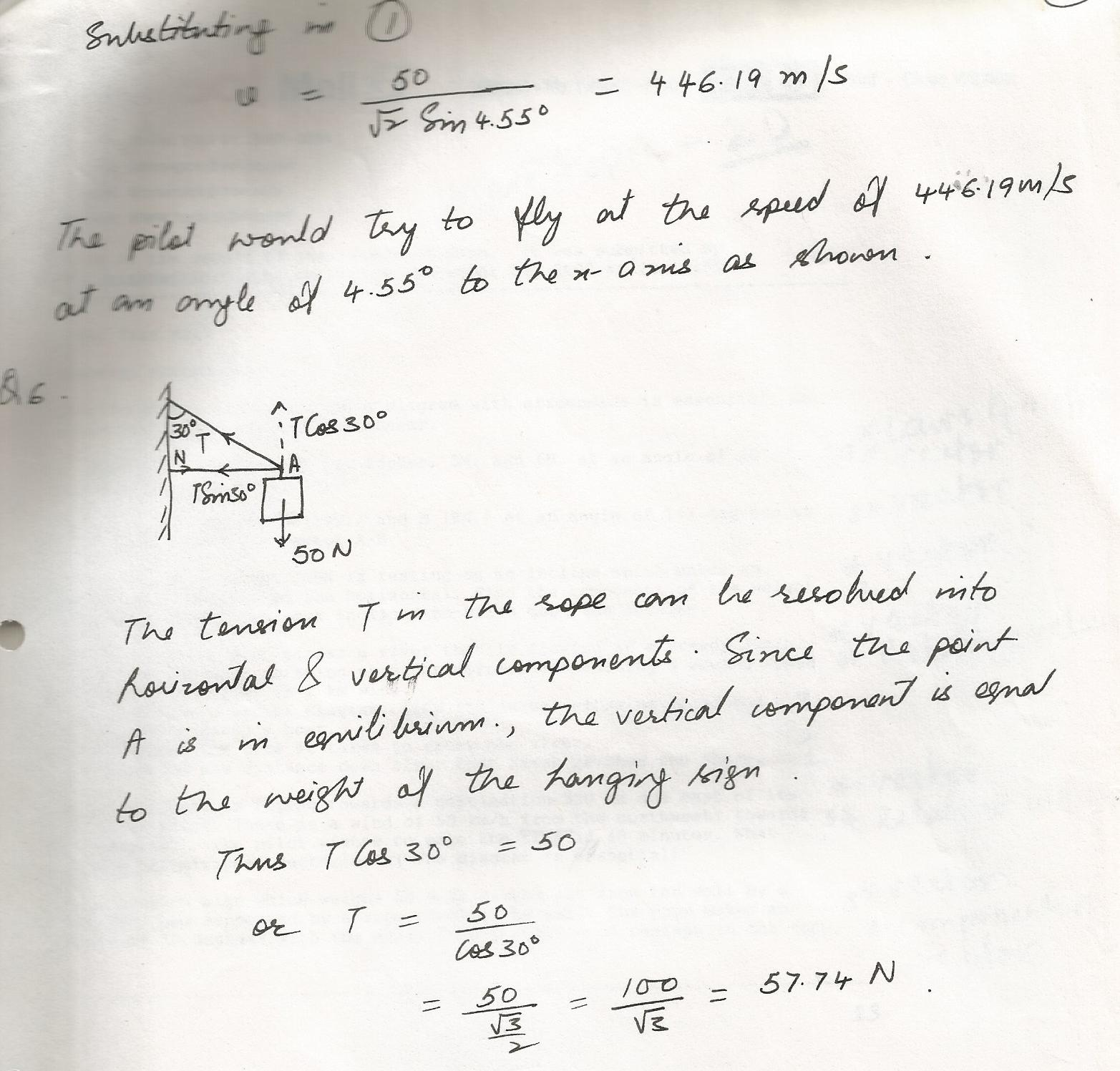7384

Physics Force & Laws Of Motion Level: Misc Level

When jumping straight down, you can be seriously injuredif you land stiff-legged. One way to avoid injury is to bend your knees upon landing to reduce the force of the impact.A 75 kg man just before contact with the ground has a speed of 6.8 m/s.

a) In a stiff- legged landing he comes to a halt in 1.8 ms. Find the average net force that acts on him during this time.

b)When he bends his knees, he comes to a halt in 0.14 s.Find the average force now.

c)During the landing, the force of the ground on the man points upward, while the force due to gravity points downward.The average net force acting on the man includes both of these force. Taking into account the directions of these forces, find the force of the ground on the man in parts (a) and (b).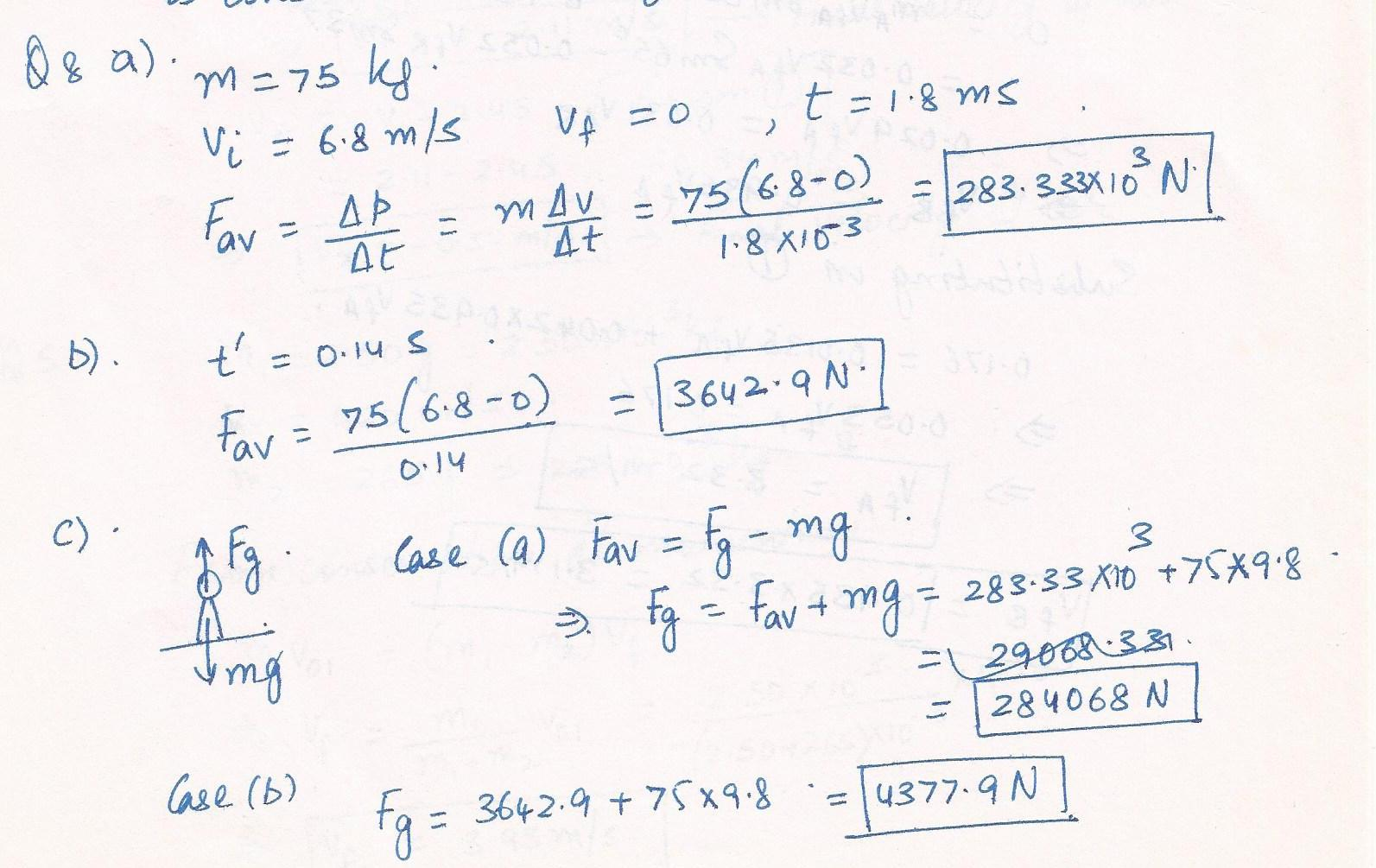7378

Physics Force & Laws Of Motion Level: Misc Level

A stream of water strikes a stationary turbine blade horizontally, as the drawing illustrates. The incident water stream has a velocity of +18.0 m/s, while the exiting water stream has a velocity of -18.0 m/s. The mass of water per second that strikes the blade is 19.0 kg/s. Find the magnitude of the average force exerted on the water by the blade.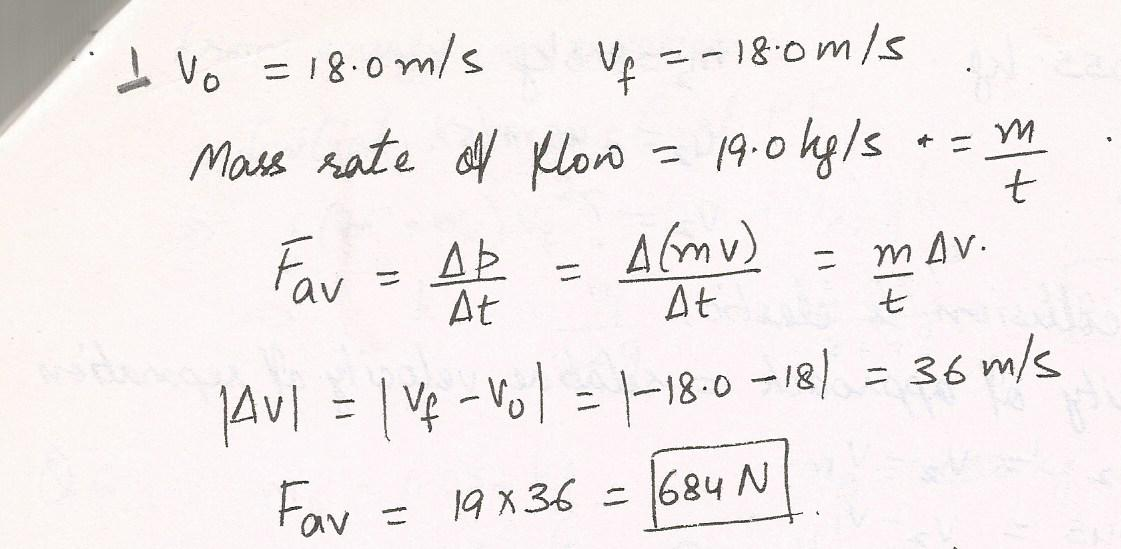7377

Physics Force & Laws Of Motion Level: Misc Level

A 2.9 kg rectangular air mattress is 2.46 m long, 0.511 m wide, and 0.172 m thick. What mass can it support in water before sinking? Answer in units of kg.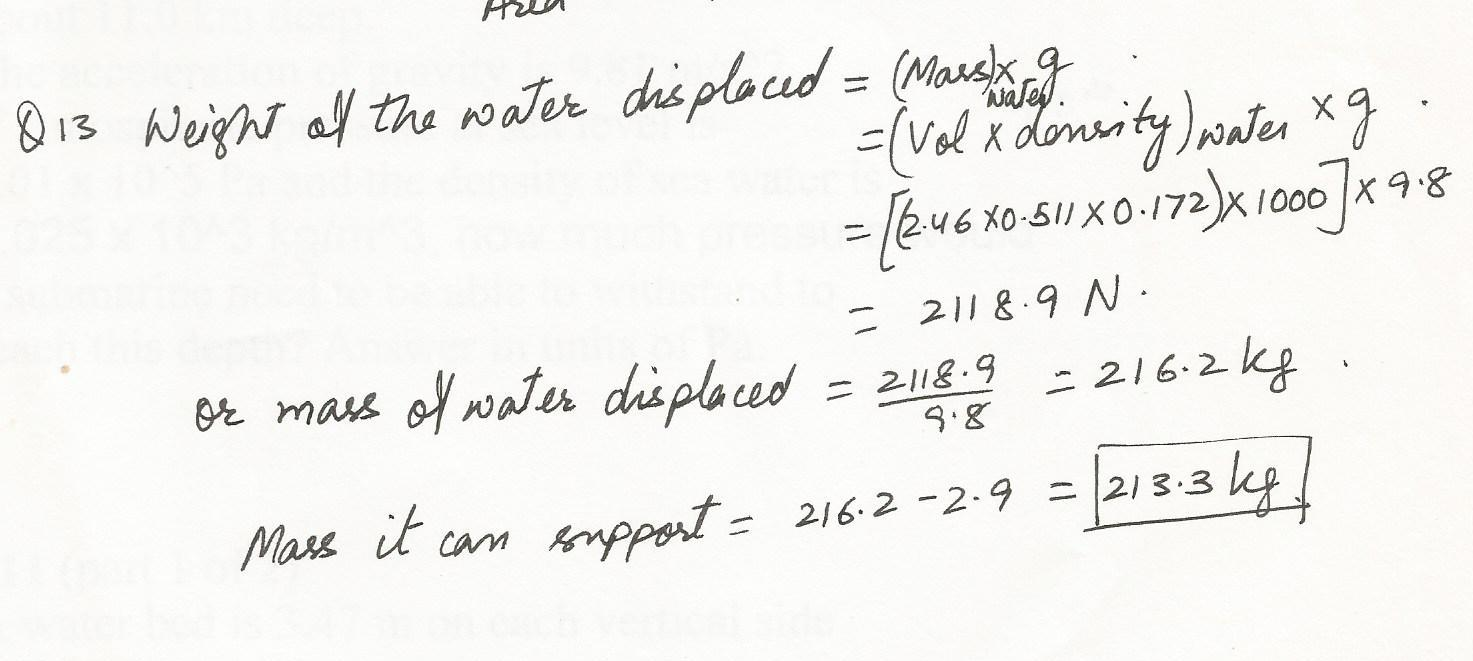7376

Physics Force & Laws Of Motion Level: Misc Level

Find the pressure exerted on the floor when the bed rests in its normal position. Assume that the entire lower surface of the bed makes contact with the floor.Answer in units of Pa.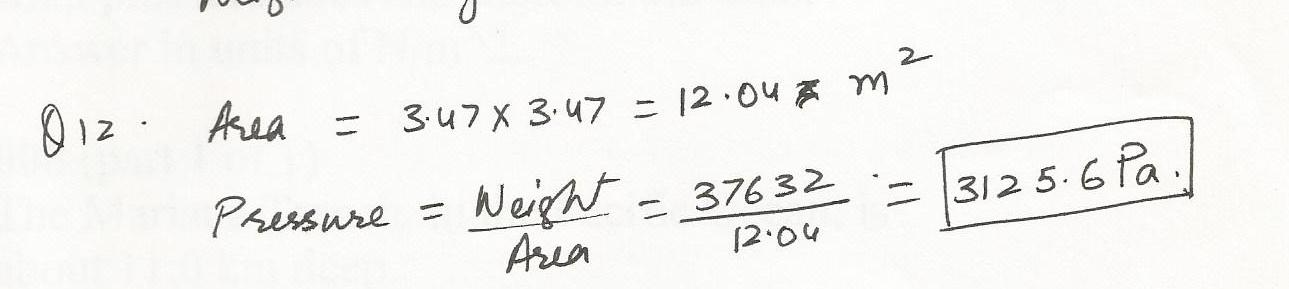7375

Physics Force & Laws Of Motion Level: Misc Level

A water bed is 3.47 m on each vertical side and 31.9 cm high.The acceleration of gravity is 9.8 m/s^2. The density of water is 1000 kg/m3. Find its weight.Answer in units of N.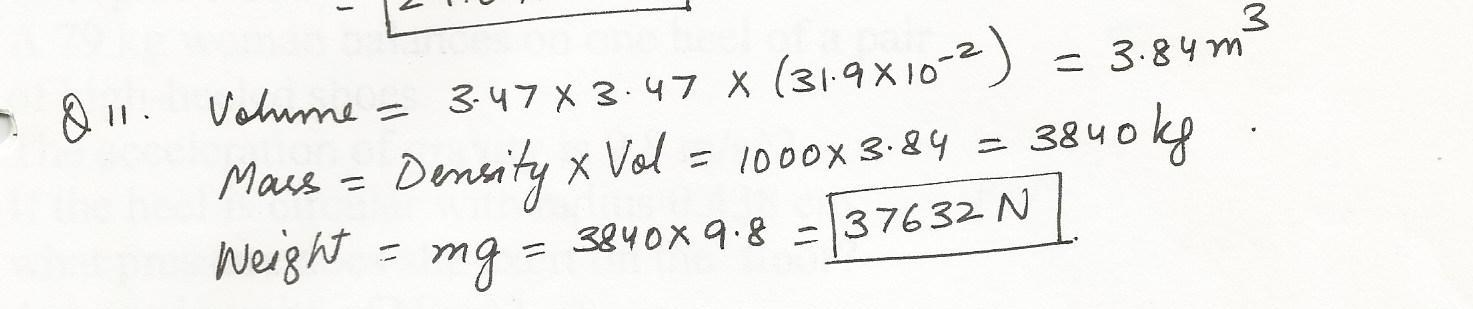7349

Physics Force & Laws Of Motion Level: Misc Level

A 0.50-kg bomb is sliding along an icy pond (frictionless surface) with a velocity of 2.0 m/s to the west The bomb explodes into two pieces. After the explosion, a 0.20 kg piece moves south at 4.0 m/s What are the components of the velocity of the 0..30 kg piece?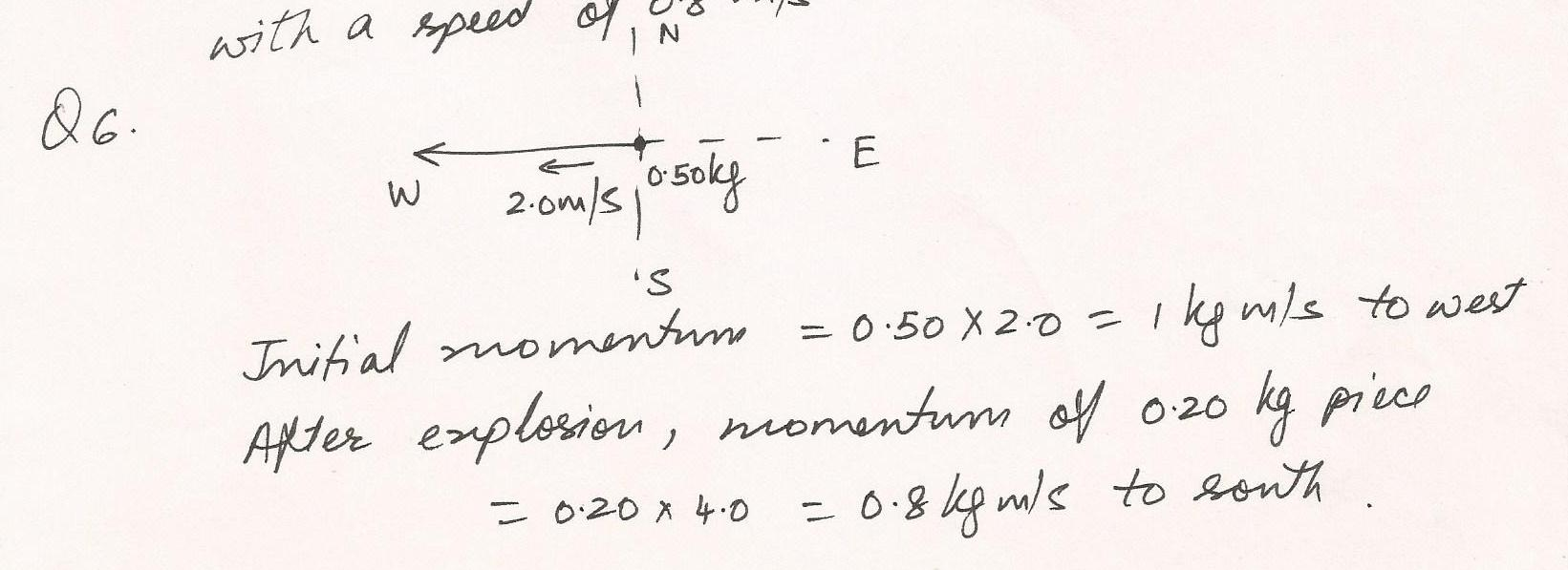7323

Physics Force & Laws Of Motion Level: Misc Level

A rope connects Boat A to Boat B.Boat A starts from rest and accelerates to a speed of 9.5 m/s in a time of 47 s. The mass of Boat B is 540 kg. Assuming a constant frictional force of 230 N on Boat B, what is the magnitude of the tension in the rope that connects the boats during the time of acceleration?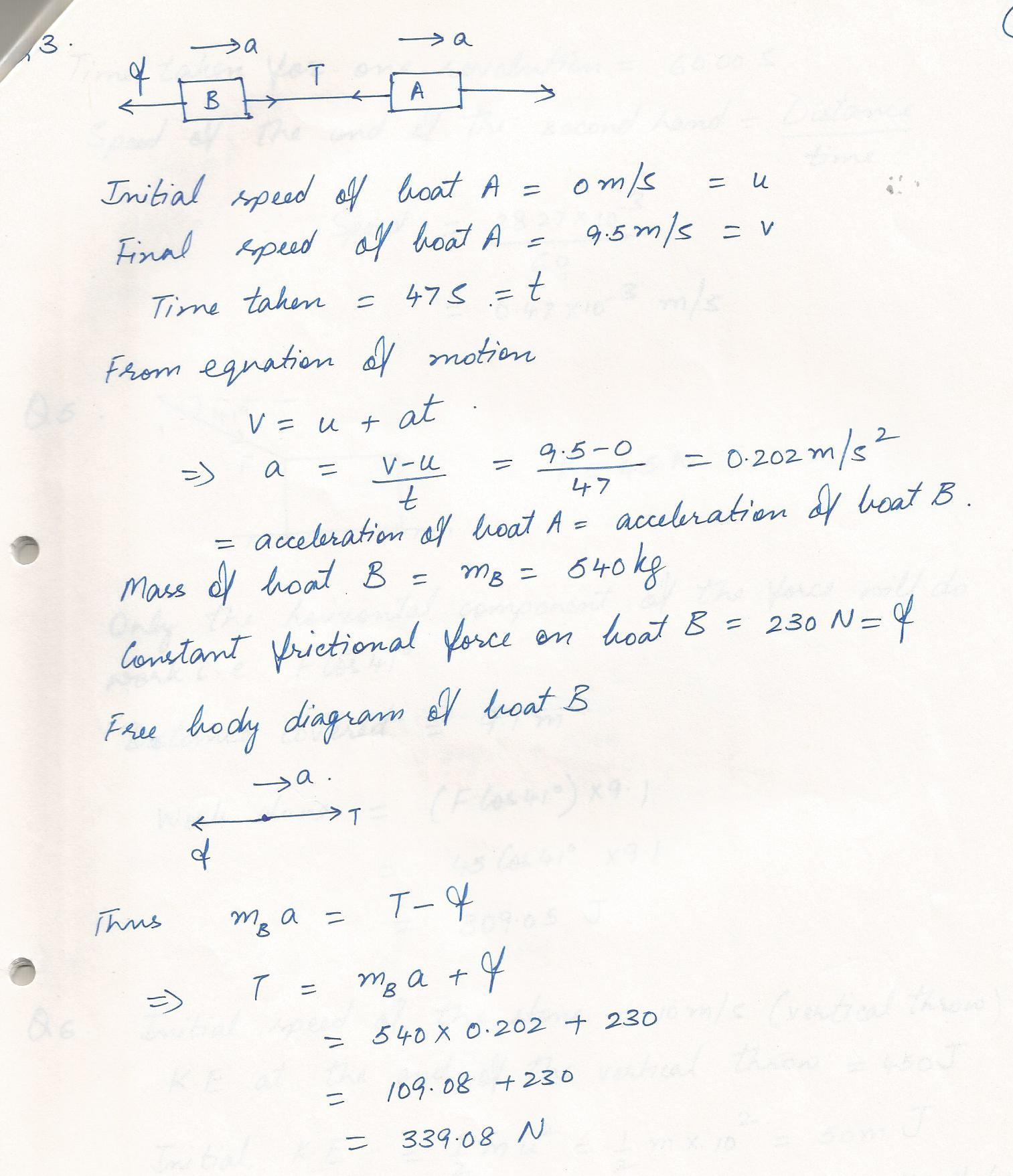7322

Physics Force & Laws Of Motion Level: Misc Level

A 20 kg object is held vertically from a fixed plank by two vertical ropes. What is the tension in each rope?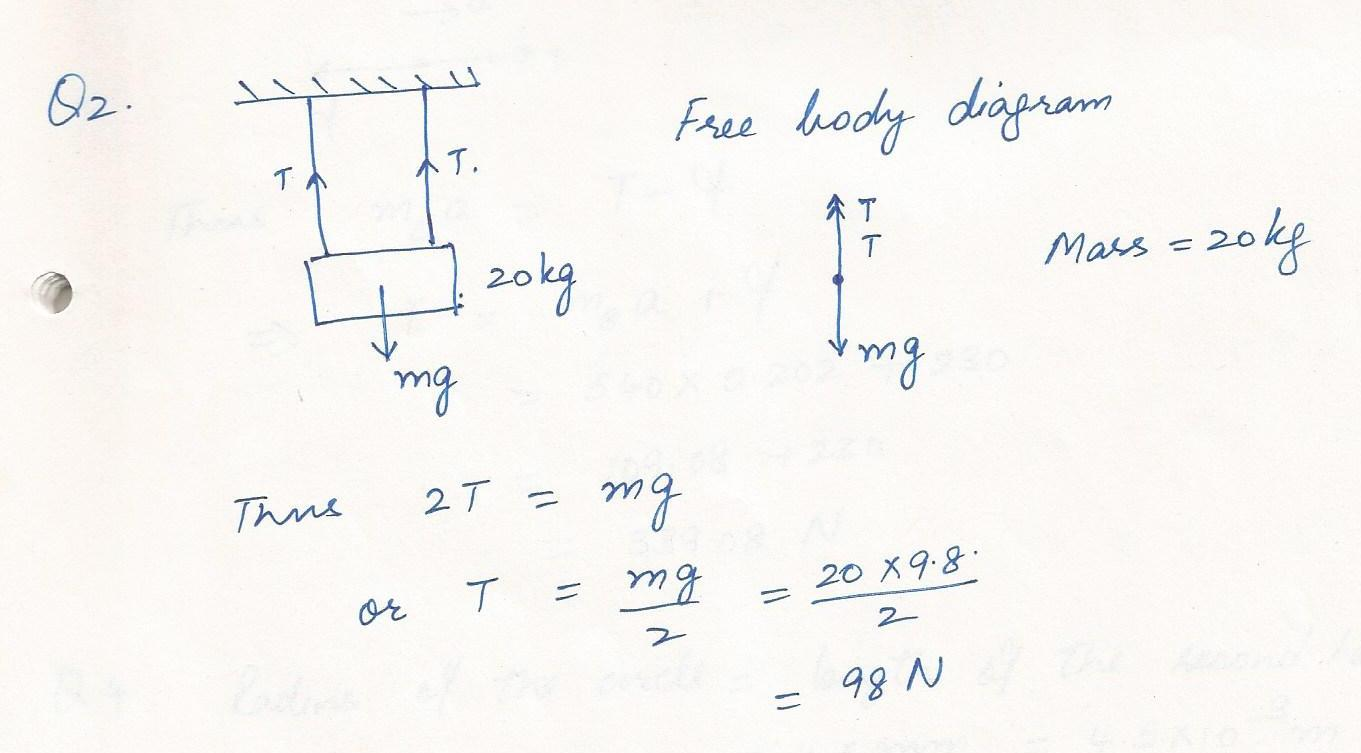7321

Physics Force & Laws Of Motion Level: Misc Level

A 10 kg block is moving at a constant speed of 6 m/s horizontally on a rough surface. If the force of friction is 20 N, approx how far will the block travel before it stops?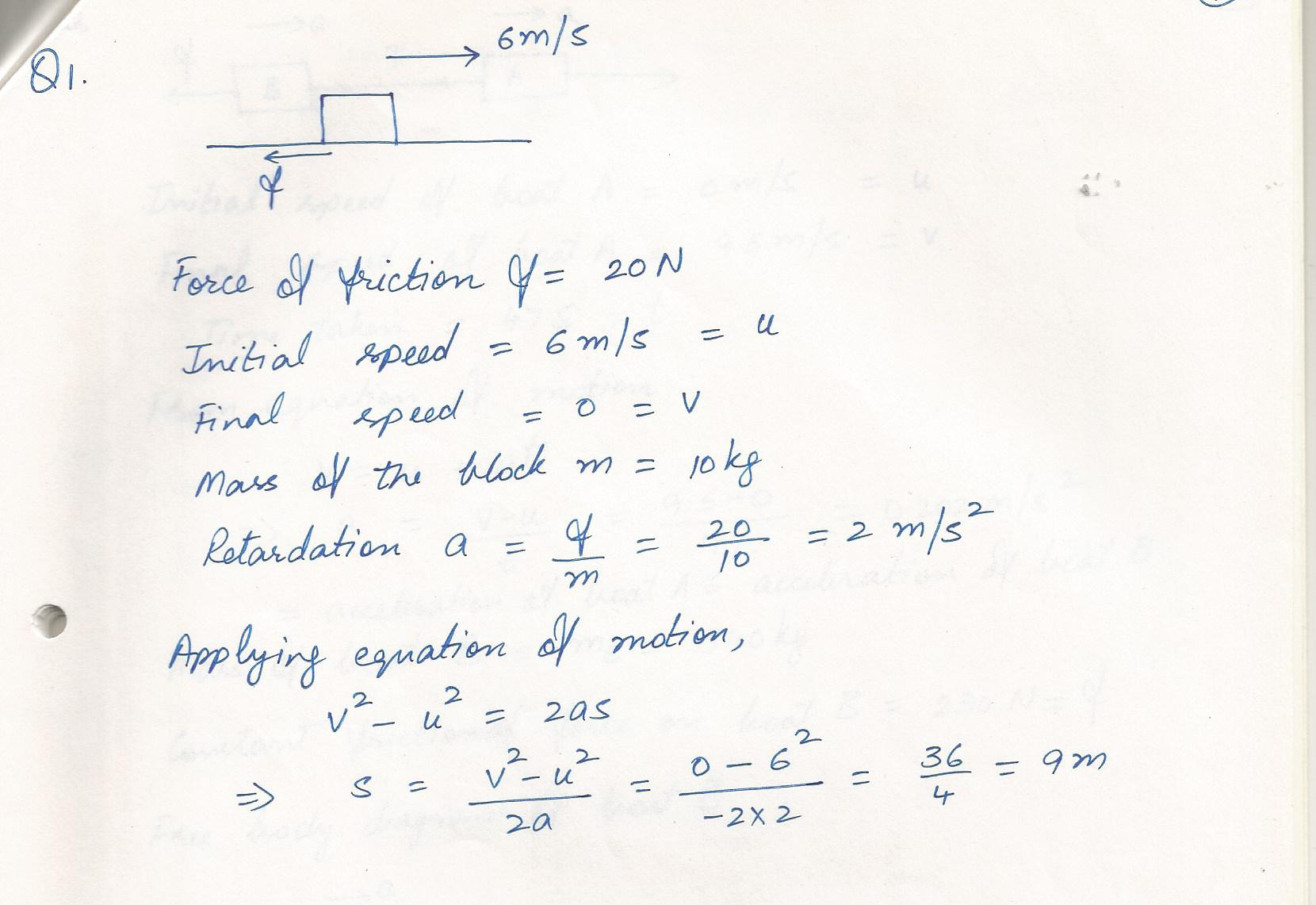7298

Physics Force & Laws Of Motion Level: Misc Level

A favorite piece of exercise equipment consists of various springs.In one exercise, a person pulls a handle grip attached to the free end of a spring to 0.80 m from the unstrained position. The other end of the spring (spring constant is 53 N/m) is held in place by the equipment from. What is the magnitude of the force that is applied to the handle grip?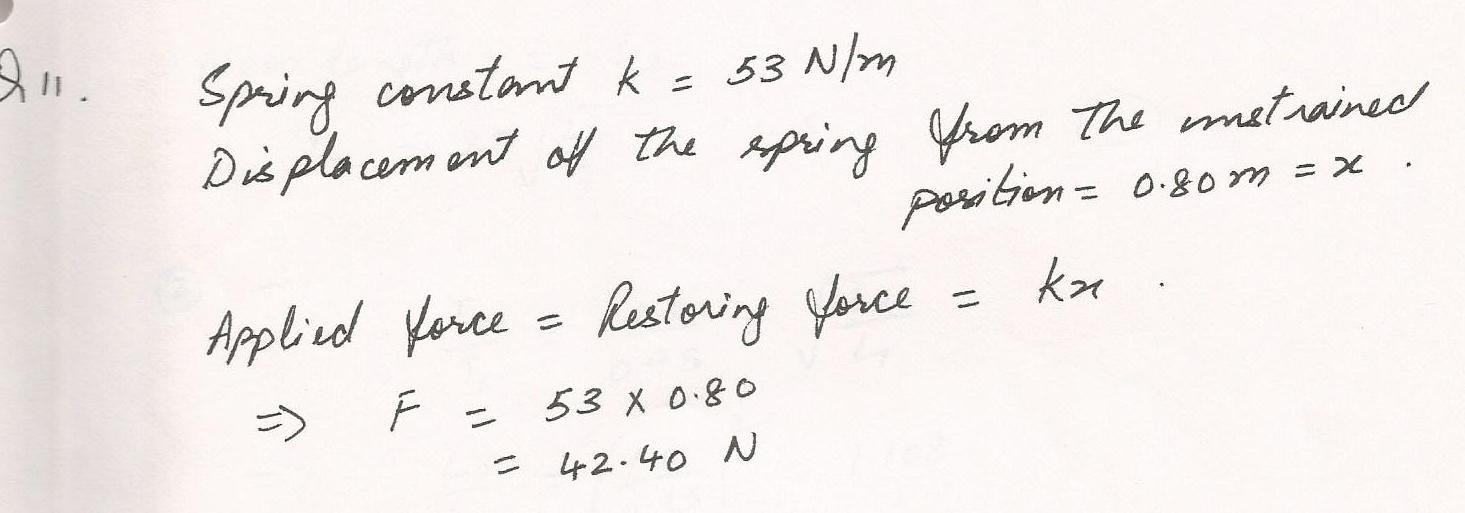7258

Physics Force & Laws Of Motion Level: Misc Level

Magnet A has twice the magnetic field strength of magnet B, and at a certain distance, it pulls on magnet B with a force of 50 N, With how much force, then does magnet B, pull on magnet A?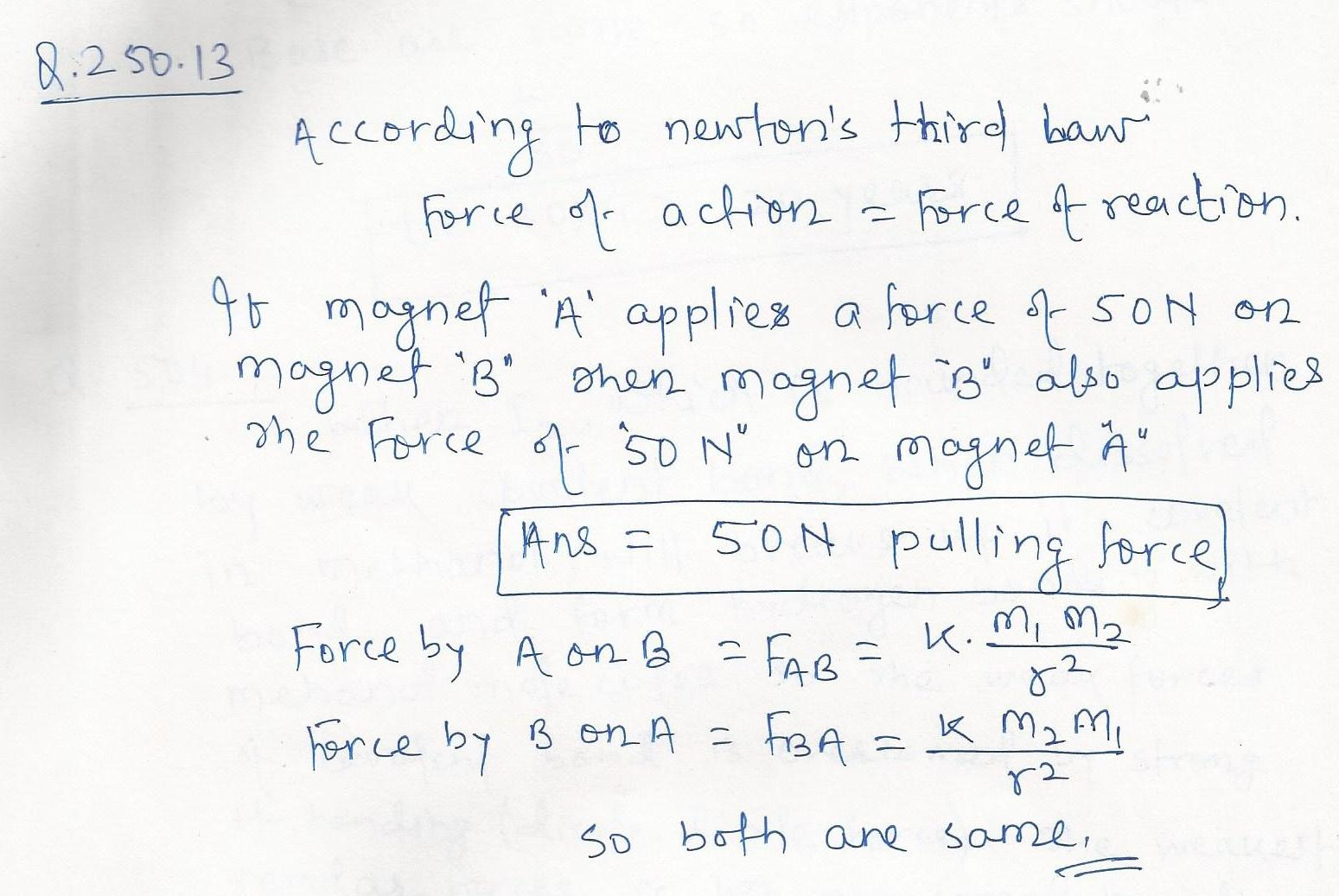7228

Physics Force & Laws Of Motion Level: Misc Level

A 3.0-g bullet traveling at 350 m/s hits a tree and slows down uniformly to a stop while penetrating a distance of 12 cm into the tree,s trunk. What was the force exerted on the bullet in bringing it rest?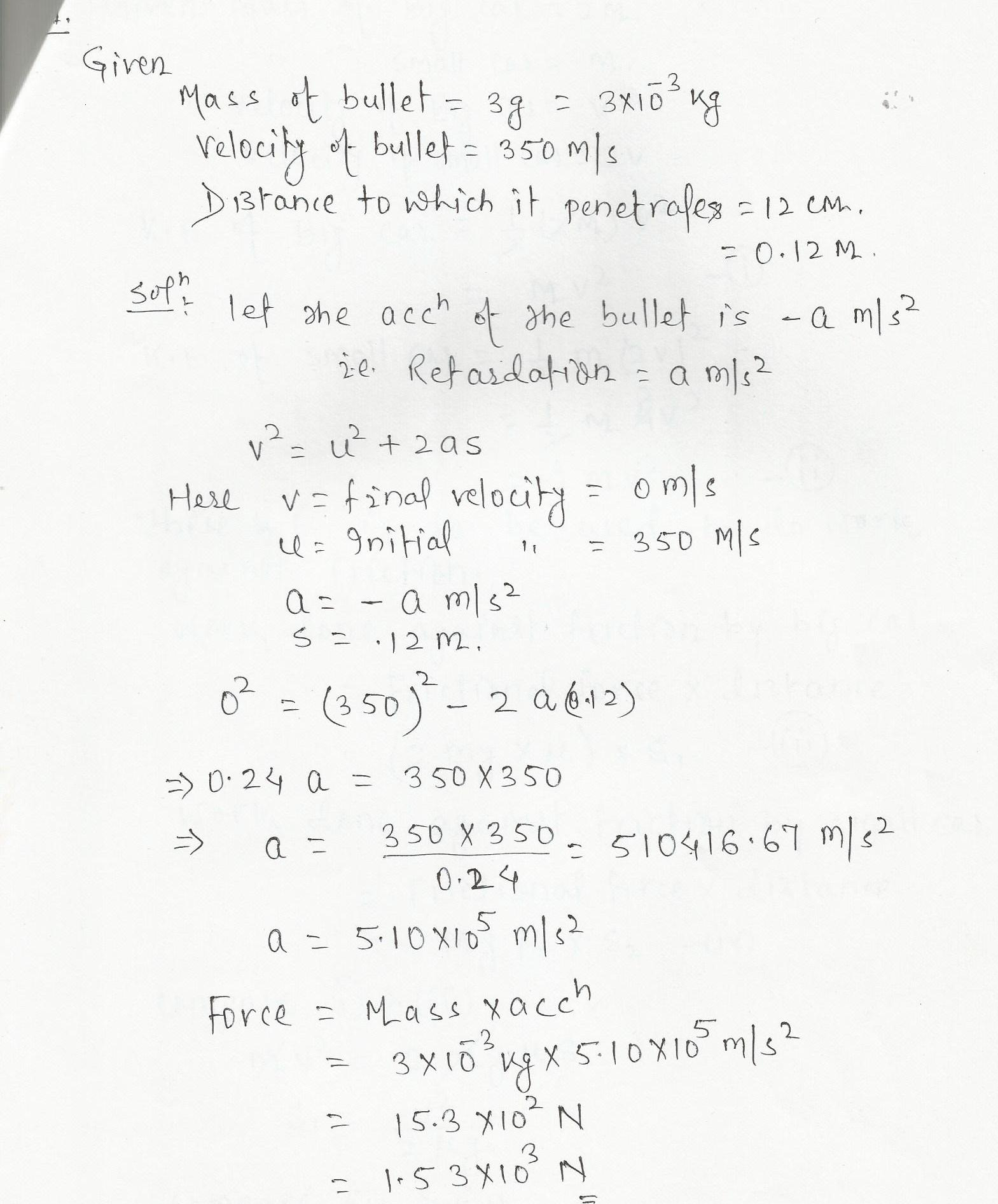6902

Physics Force & Laws Of Motion Level: Misc Level

Block A, with a mass of 10 kg, rests on a 30% incline ,The coefficient of kinetic friction is 0.20. The attached string is parallel to the incline and passes over a massless, frictionless pulley at the top. Block B, with a mass of 3.0 kg, is attached to the dangling end of the string . Find the acceleration of B (magnitude and direction);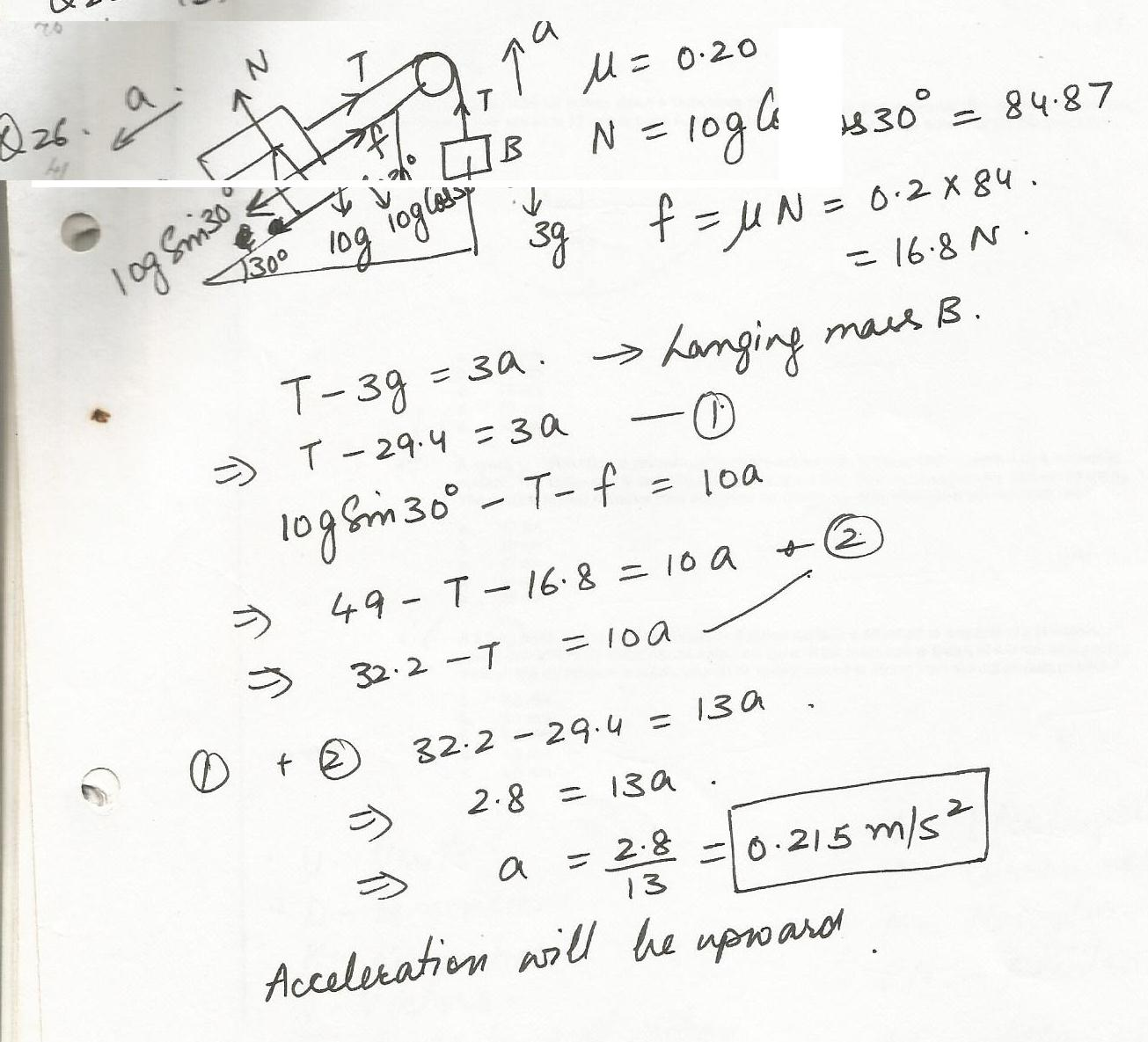Displaying 31-45 of 709 results.